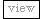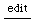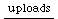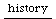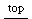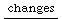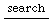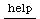## too many expatriates

JMcC( This error doesn't happen anymore. In previous versions of SC3d5 there were some extremely clever ways to share objects between the two virtual machines. As with most extremely clever solutions, it was a real headache, so I decided to go the clean way of full separation of the object spaces. )JMcC
```//how to create this error:

???

//error:

€ ERROR: Too many expatriates.
ERROR:
Primitive '_SendMsg' failed.

Failed.
Instance of Main {    (08F51670, gc=F1C, fmt=00, flg=00, set=03)
instance variables 
classVars : instance of Array (08E56420, size=1148, set=0B)
interpreter : instance of Interpreter (08F517F0, size=0, set=05)
mainThread : instance of Thread (08F516D0, size=0, set=05)
processID : Integer 0
expatriatesSent : instance of Array (08E5A860, size=256, set=08)
expatriatesReached : instance of Array (08E5B090, size=3, set=08)
}
CALL STACK:
Object::primitiveFailed
arg this =
Process::sendMsg
arg this =
arg toProcessID = 1
arg args = [*3]
Function::send
arg this =
arg args = [*1]
< FunctionDef in closed FunctionDef >
arg x = 0.266602
arg y = 0.625000
ClickView::mouseDown
arg this =
arg where =
arg event =
TraceView::mouseDown
arg this =
arg where =
arg event =
< FunctionDef in Method View::mouseDown >
arg item =
var tracker = nil
ArrayedCollection::reverseDo
arg this = [*2]
arg function =
var i = 1
var j = 0
...

//solution:

???

```